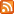From Small Bushbaby, 1 Week ago, written in Python.
This paste is a reply to Untitled from Diminutive Cat - view diff
Embed
Hits: 211. import math
2.
3. if __name__ == '__main__':
4.     target = int(input("Enter phone number without leading 0:\n"))
5.
6.     current_value = 7  # initializes with 7 press
7.     number_of_button_presses = 1  # initialized with press
8.     number_of_seven_presses = 1
9.     number_of_square_presses = 0
10.     number_of_times3_presses = 0
11.     number_of_2_presses = 0
12.
13.     while current_value ** 2 < target:
14.         current_value = current_value ** 2
15.         number_of_button_presses += 1
16.         number_of_square_presses += 1
17.         print(current_value, number_of_button_presses)
18.
19.     while current_value * 3 < target:
20.         current_value = current_value * 3
21.         number_of_button_presses += 1
22.         number_of_times3_presses += 1
23.         print(current_value, number_of_button_presses)
24.
25.     n = (target - current_value) / 7
26.     current_value += math.floor(n) * 7
27.     number_of_button_presses += math.floor(n)
28.     number_of_seven_presses += math.floor(n)
29.
30.     if current_value == target:
31.         print("finished in {} presses"
32.               "\n+7 presses {}"
33.               "\n-2 presses {}"
34.               "\ntimes 3 presses {}".format(number_of_button_presses,number_of_seven_presses,number_of_2_presses,
35.                                             number_of_times3_presses))
36.         exit()
37.     else:
38.         current_value += 7
39.         number_of_seven_presses += 1
40.         number_of_button_presses += 1
41.         div = target - current_value
42.         if div % 2 == 1:
43.             current_value += 7
44.             number_of_seven_presses += 1
45.             number_of_button_presses += 1
46.
47.     while current_value != target:
48.         current_value -= 2
49.         number_of_2_presses += 1
50.         number_of_button_presses += 1
51.     print("finished in {} presses"
52.           "\nsquare presses: {}"
53.           "\n+7 presses: {}"
54.           "\n-2 presses: {}"
55.           "\ntimes 3 presses: {}".format(number_of_button_presses,number_of_square_presses, number_of_seven_presses, number_of_2_presses,
56.                                         number_of_times3_presses))
57.

# Replies to Re: UntitledTitle Name Language When
Re: Re: Untitled Sweltering Rhinoceros python 1 Week ago.